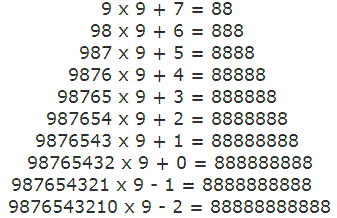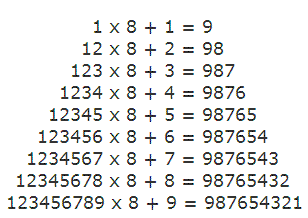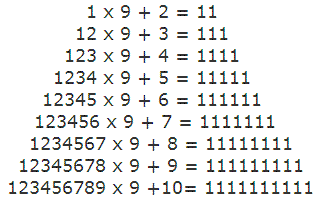### Beauty of Math

Look at these brilliant calculations!This symmetry is brilliant too!Now, take a look at this...

From a strictly mathematical viewpoint:

What equals 100%?
What does it mean to give MORE than 100%?
Ever wonder about those people who say they are giving more than 100%?
We have all been in situations where someone wants you to GIVE OVER 100% .

Here's a little mathematical formula that might help answer these questions.

If:
A B C D E F G H I J K L M N O P Q R S T U V W X Y Z

Is represented as:
1 2 3 4 5 6 7 8 9 10 11 12 13 14 15 16 17 18 19 20 21 22 23 24 25 26

If:
H-A-R-D-W-O-R-K
8+1+18+4+23+15+18+11 = 98%

And:
K-N-O-W-L-E-D-G-E
11+14+15+23+12+5+4+7+5 = 96%

But:
A-T-T-I-T-U-D-E
1+20+20+9+20+21+4+5 = 100%

THEN,
look how far the love of God will take you:

L-O-V-E-O-F-G-O-D
12+15+22+5+15+6+7+15+4 = 101%

Therefore, one can conclude with mathematical certainty that:

While Hard Work and Knowledge will get you close, and Attitude will get you there,
it's the Love of God that will put you over the top!